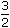# Aptitude - Partnership

### Exercise :: Partnership - Data Sufficiency 2

Each of the questions given below consists of a question followed by three statements. You have to study the question and the statements and decide which of the statement(s) is/are necessary to answer the question.

1.

 How much did Rohit get as profit at the year-end in the business done by Nitin, Rohit and Kunal? I. Kunal invested Rs. 8000 for nine months, his profit wastimes that of Rohit's and his investment was four times that of Nitin. II. Nitin and Rohit invested for one year in the proportion 1 : 2 respectively. III. The three together got Rs. 1000 as profit at the year end.

 A. Only I and II B. Only I and III C. Question cannot be answered even with the information in all the three statements. D. All I, II and III E. None of these

Explanation:

I and II give:

K = Rs. (8000 x 9) for 1 month = Rs. 72000 for 1 month.

 N = Rs.1 x 8000 x 12for 1 month = Rs. 24000 for 1 month. 4

R = Rs. 48000 for 1 month.K : N : R = 72000 : 24000 : 48000 = 3 : 1 : 2.

III gives, total profit = Rs. 1000.Rohit's share = Rs.1000 x 2= Rs. 333 1 6 3Correct answer is (D).

2.

 What is R's share of profit in a joit venture? I. Q started business investing Rs. 80,000. II. R joined him after 3 months. III. P joined after 4 months with a capital of Rs. 1,20,000 and got Rs. 6000 as his share profit.

 A. All I, II and III B. I and III only C. II and III only D. Even with all I, II and III, the answer cannot be arrived at E. None of these

Explanation:

From I, II and III, we get P : Q : R = (120000 x 8) : (80000 x 12) : (x x 9).

Since R's investment is not given, the above ratio cannot be give.Given data is inadequate.